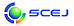## \$B9V1i%W%m%0%i%`!J%;%C%7%g%sJL!K(B

### \$BN3;R!&N.BN%W%m%;%9(B

#### A114-A119

\$B:G=*99?7F|;~!'(B2012-07-17 17:08:32
\$B9V1i(B
\$B;~9o(B
\$B9V1i(B
\$BHV9f(B
\$B9V1iBjL\!?H/I=\$B%-!<%o!<%I(B\$BJ,N`(B
\$BHV9f(B
\$B\$BHV9f(B
A\$B2q>l(B \$BBh(B1\$BF|(B
(13:20\$B!A(B14:20)\$B!!(B(\$B:BD9(B \$B>.NS(B \$BBgM4(B)
13:20\$B!A(B 13:40A114\$B%O!<%U!&%>!<%s7?(BFZ\$BFbBPN.9=B\$\$K5Z\$\\$929EY:9!&G;EY:9%^%i%s%4%KBPN.\$N1F6A(B
(\$BN0Bg9)(B) (\$B@5(B)\$B?e8}(B \$B>0(B \$B!&(B \$B574V(B \$B8g(B \$B!&(B (\$B:eBg1!4p9)(B) (\$B@5(B)\$B9bLZ(B \$BMNJ?(B \$B!&(B \$B!{(B(\$B@5(B)\$B2,Ln(B \$BBYB'(B
Mrangoni convection
Floating Zone
Numerical simulation
2-a15
13:40\$B!A(B 14:00A115\$B%^%\$%/%mN.O)Fb\$K\$*\$1\$k8r8_N.(B-\$B3&LL3h@-:^\$N1F6A(B-
(\$B2,M}Bg1!(B) \$B!{(B(\$B3X(B)\$B>>Ln(B \$B905.(B \$B!&(B (\$B2,M}Bg9)(B) (\$B@5(B)\$BJ?Ln(B \$BGnG7(B \$B!&(B (\$B@5(B)\$B2,K\(B \$BD>9'(B
microchannel
alternating flow
surfactant
2-a76
14:00\$B!A(B 14:20A116\$BEE5\$Dq93%H%b%0%i%U%#\$K\$h\$k6k7AMF4oFb2D;k2=\$N8!F\$(B
(IHI) \$B!{0l>r(B \$B7{L@(B \$B!&(B \$B>>Ln(B \$B?-2p(B \$B!&(B \$B \$B!&(B \$BFJLZ(B \$BA19n(B \$B!&(B (\$B2#9qBg1!(B) (\$B@5(B)\$B;03Q(B \$BN4B@(B \$B!&(B (\$B@5(B)\$B?N;V(B \$BOBI'(B \$B!&(B (\$B@5(B)\$B>e%N;3(B \$B<~(B
Electrical resistance tomography
Image reconstruction
Glass melter
2-e75
(14:20\$B!A(B15:20)\$B!!(B(\$B:BD9(B \$B2,Ln!!BYB'(B)
14:20\$B!A(B 14:40A117\$B3IYBAeFb\$K\$*\$1\$k3IYB1U(B-\$BNd5Q%3%\$%k4V\$NG.EAC#(B
(\$B;37ABg1!M}9)(B) \$B!{(B(\$B3X(B)\$B@iMU(B \$BN \$B!&(B (\$B@5(B)\$BLg3p(B \$B=( \$B!&(B (\$B@5(B)\$B86ED(B \$B1QFs(B \$B!&(B (\$B@5(B)\$B7*;3(B \$B2mJ8(B
Agitated vessel
Heat transfer
Cooling coil
2-b20
14:40\$B!A(B 15:00A118\$B8G1UYxYB\$K\$*\$1\$kAeJIG.EAC#(B
(\$B;37ABgM}9)(B) \$B!{(B(\$B3X(B)\$B]/0f(B \$BM%M4(B \$B!&(B (\$B@5(B)\$BLg3p(B \$B=( \$B!&(B (\$B@5(B)\$B86ED(B \$B1QFs(B \$B!&(B (\$B@5(B)\$B7*;3(B \$B2mJ8(B
Solid-Liquid Agitation
Heat Transfer
Particle Size
2-b60
15:00\$B!A(B 15:20A119\$B1UCf4%AgK!\$K\$h\$k%\$%L%j%sHyN3;R\$ND4@=\$H7ABV@)8f(B
(\$BElM}Bg9)(B) \$B!{(B(\$B@5(B)\$B9b66(B \$BCR51(B \$B!&(B \$B7*86(B \$BBs:H(B \$B!&(B \$B3^86(B \$BN4;N(B \$B!&(B \$B=a4V(B \$B?r;V(B \$B!&(B (\$B@5(B)\$B>.NS(B \$BBgM4(B \$B!&(B (\$B@5(B)\$B>1Ln(B \$B8|(B \$B!&(B (\$B@5(B)\$BBgC](B \$B>!?M(B
inulin
nano-particle
crystallization
12-g99

\$B9V1i%W%m%0%i%`(B
\$B2=3X9)3X2q2#IMBg2q(B2012(C) 2012 \$B8x1W
Most recent update: 2012-07-17 17:08:32
E-mail: inquiry-kt2012www3.scej.org
This page was generated byeasp 2.28; proghtml 2.28c (C)1999-2011 kawase